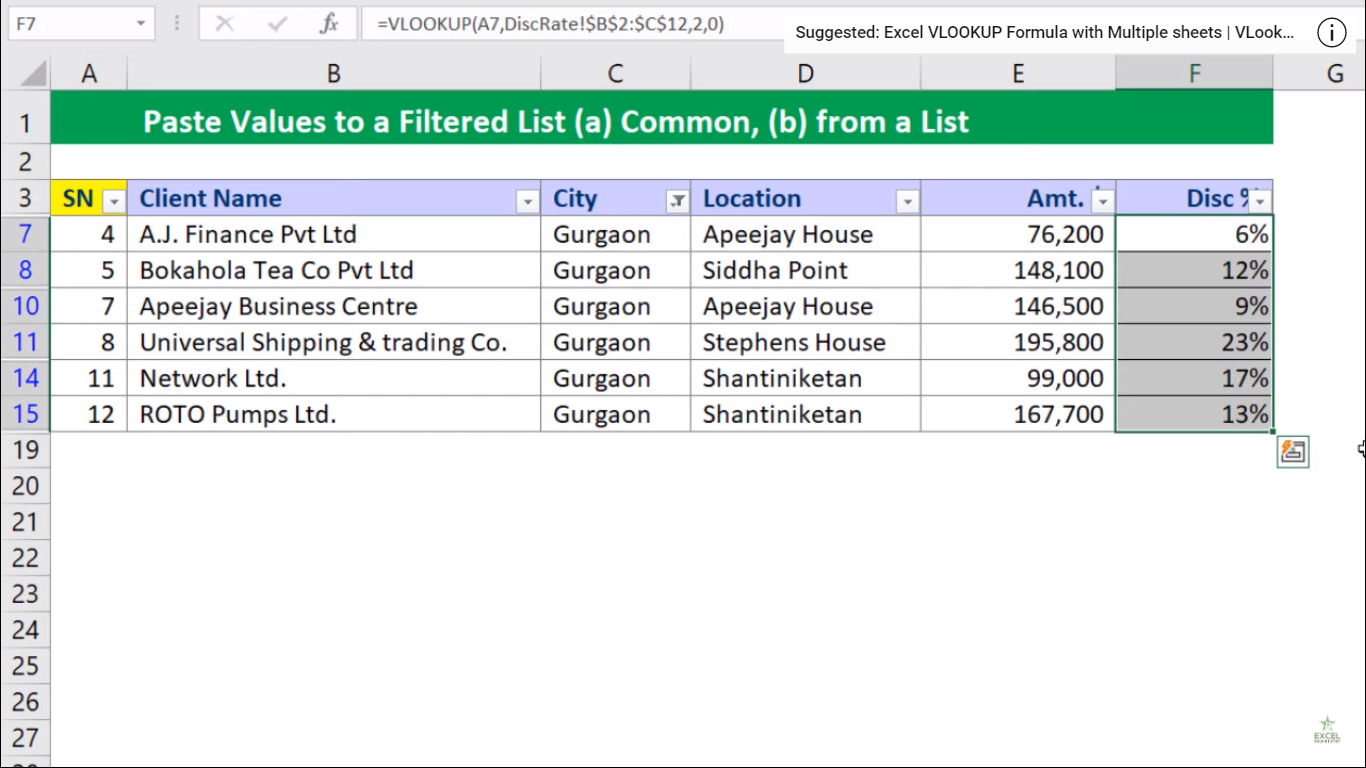# Excel Paste Values to a Filtered ListIn this tutorial, we will learn about How to Paste Values to a Filtered List with 2 things:

1. Common Values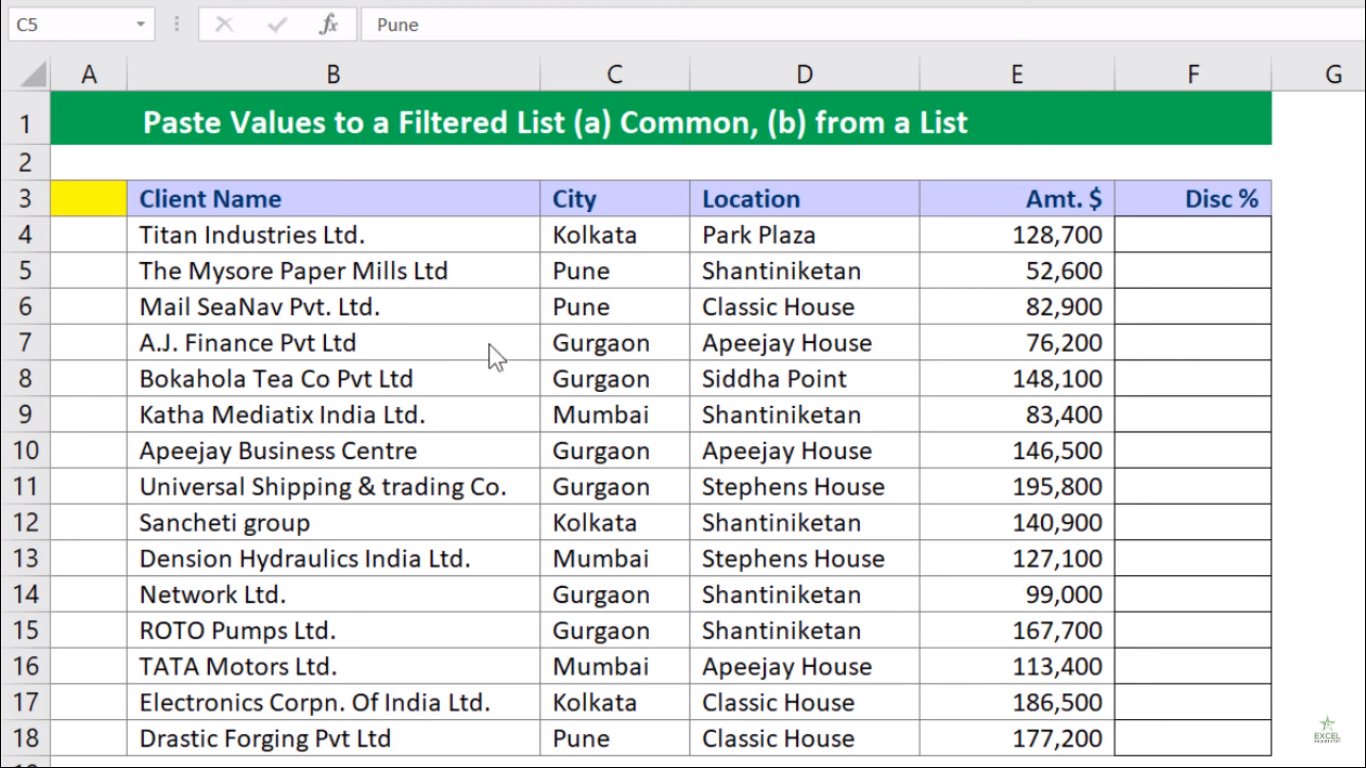2. From a list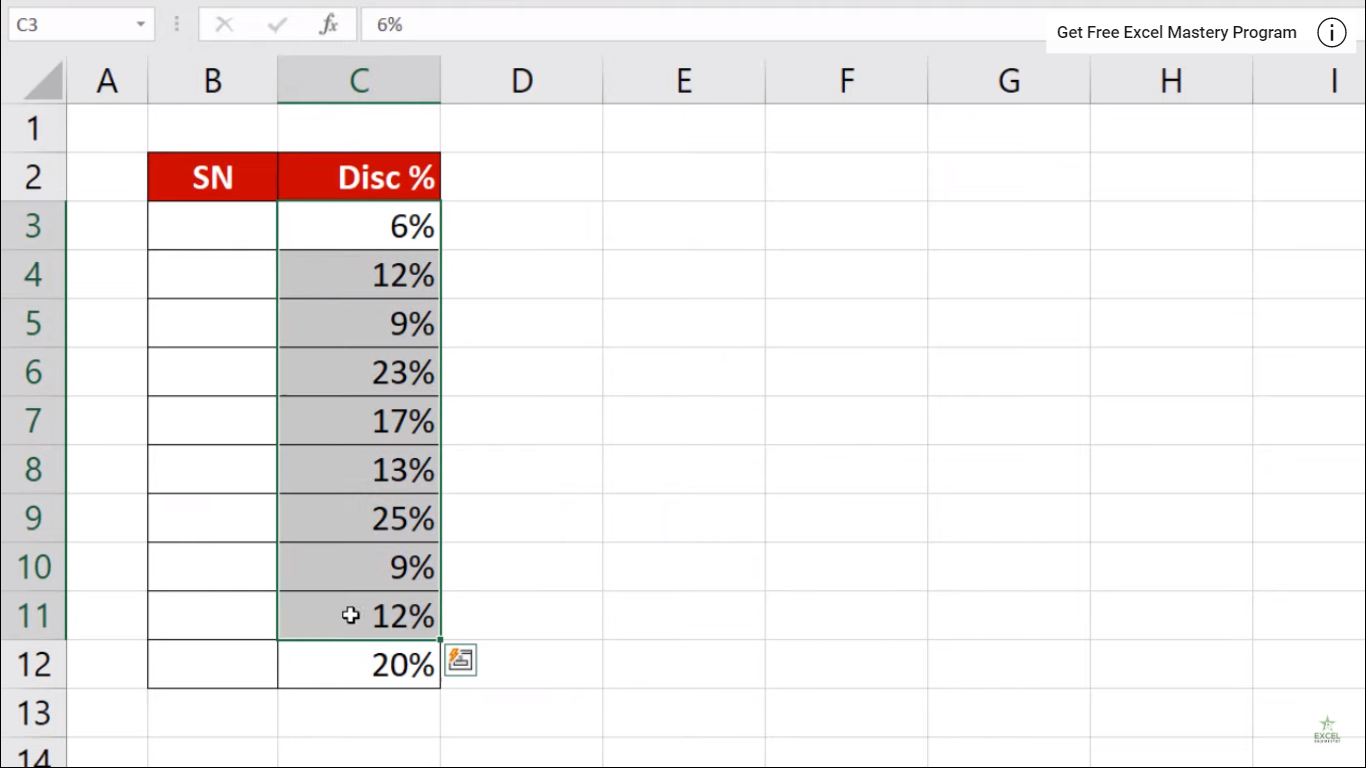1. Common Values

Assume that you have a table and you do the following things:

1. Choose the Entire Data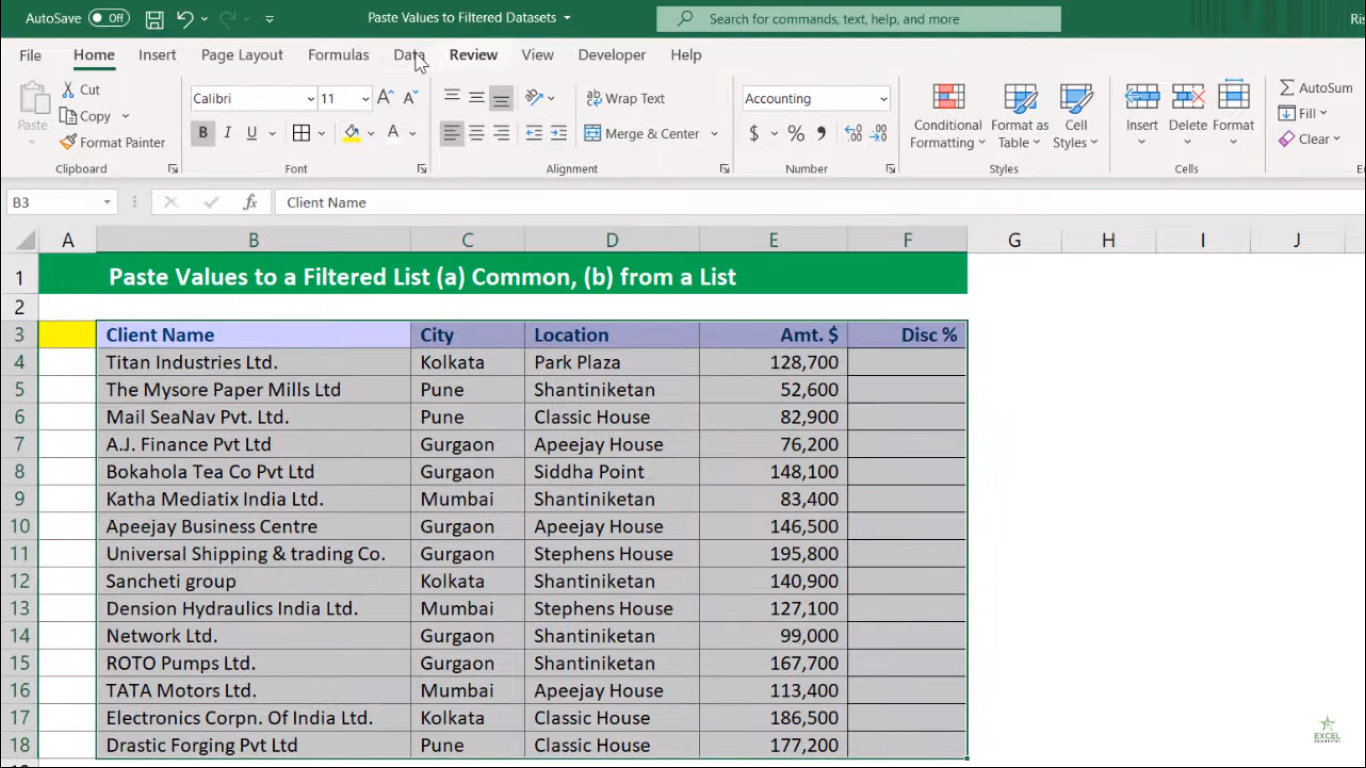2. Apply the Filter by going to Data Tab > Filter Button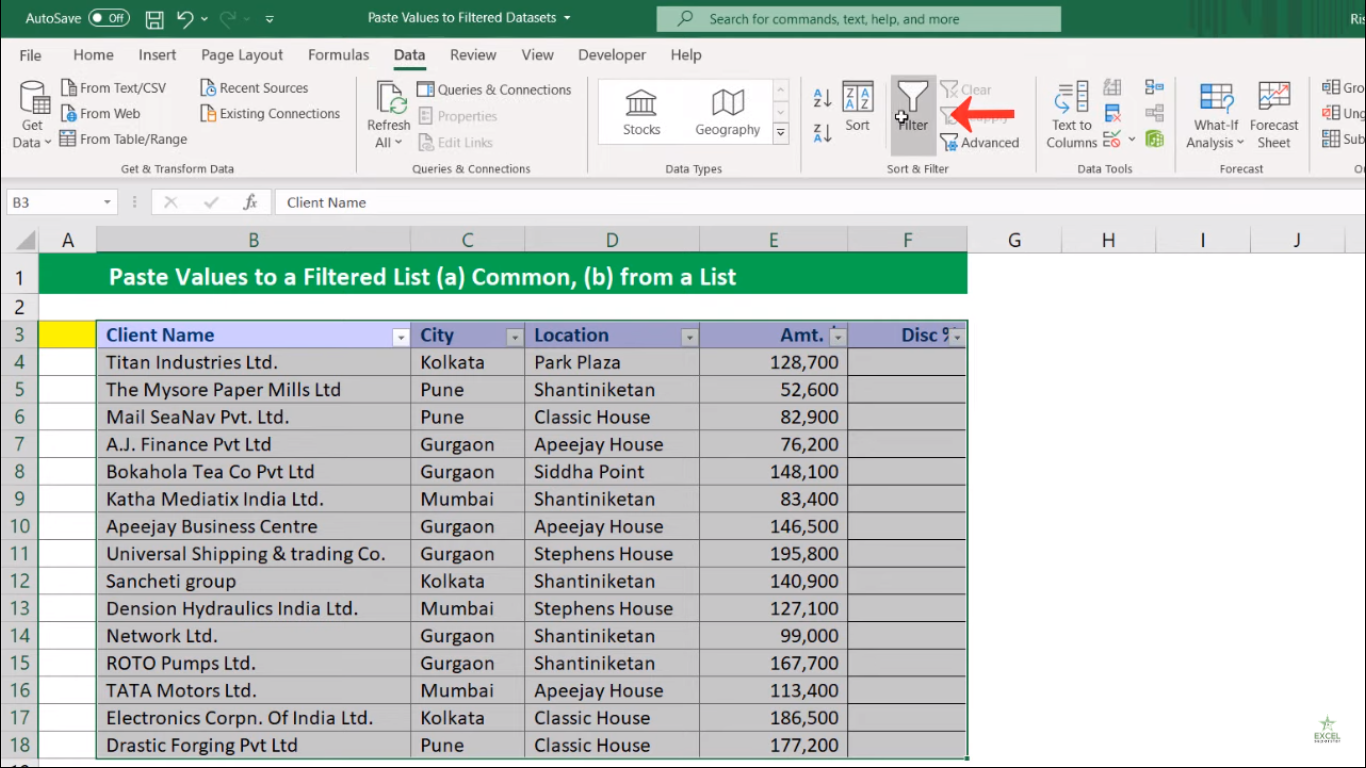3. Select the Gurgaon City values from the City Column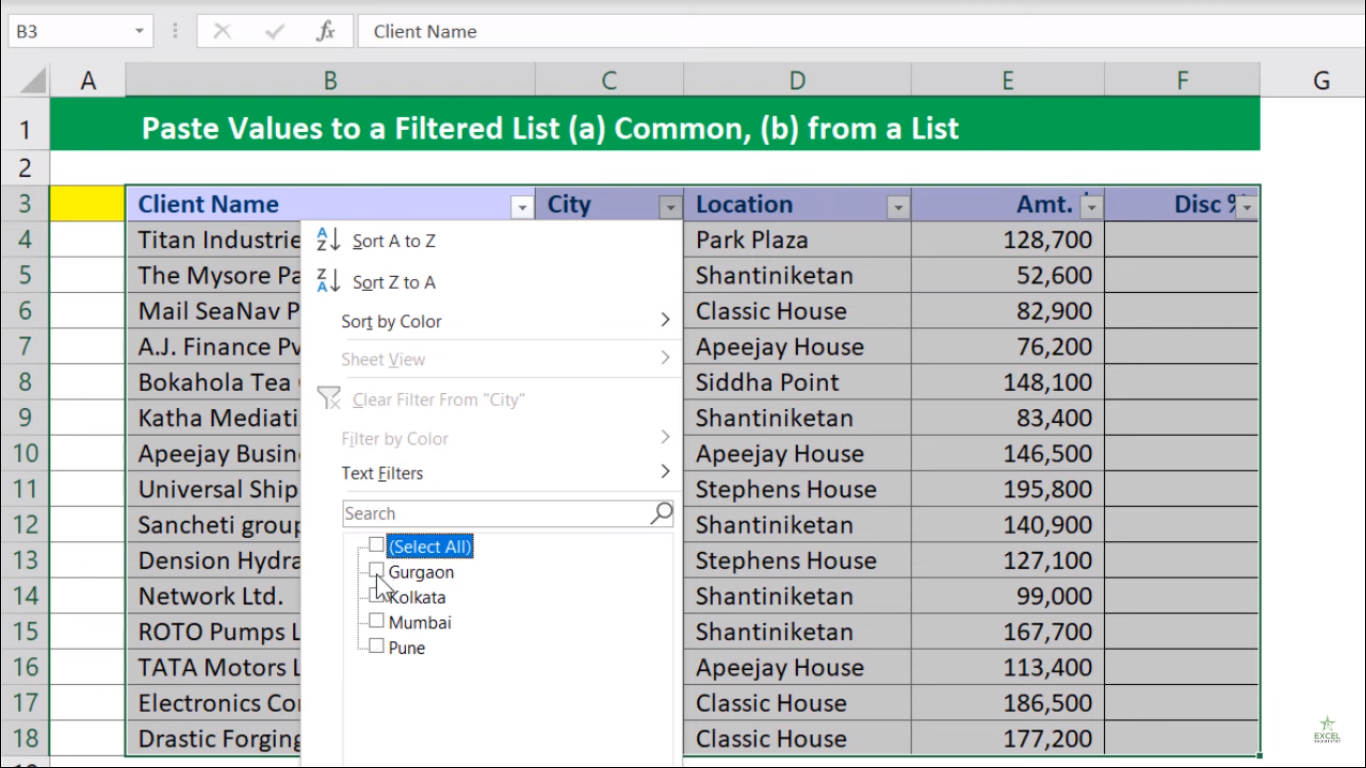Now what you have to do is you have to keep a Discount Percentage of 10% from all the Gurgaon City Values and apply it in the Discount Column. So here’s how you can do it: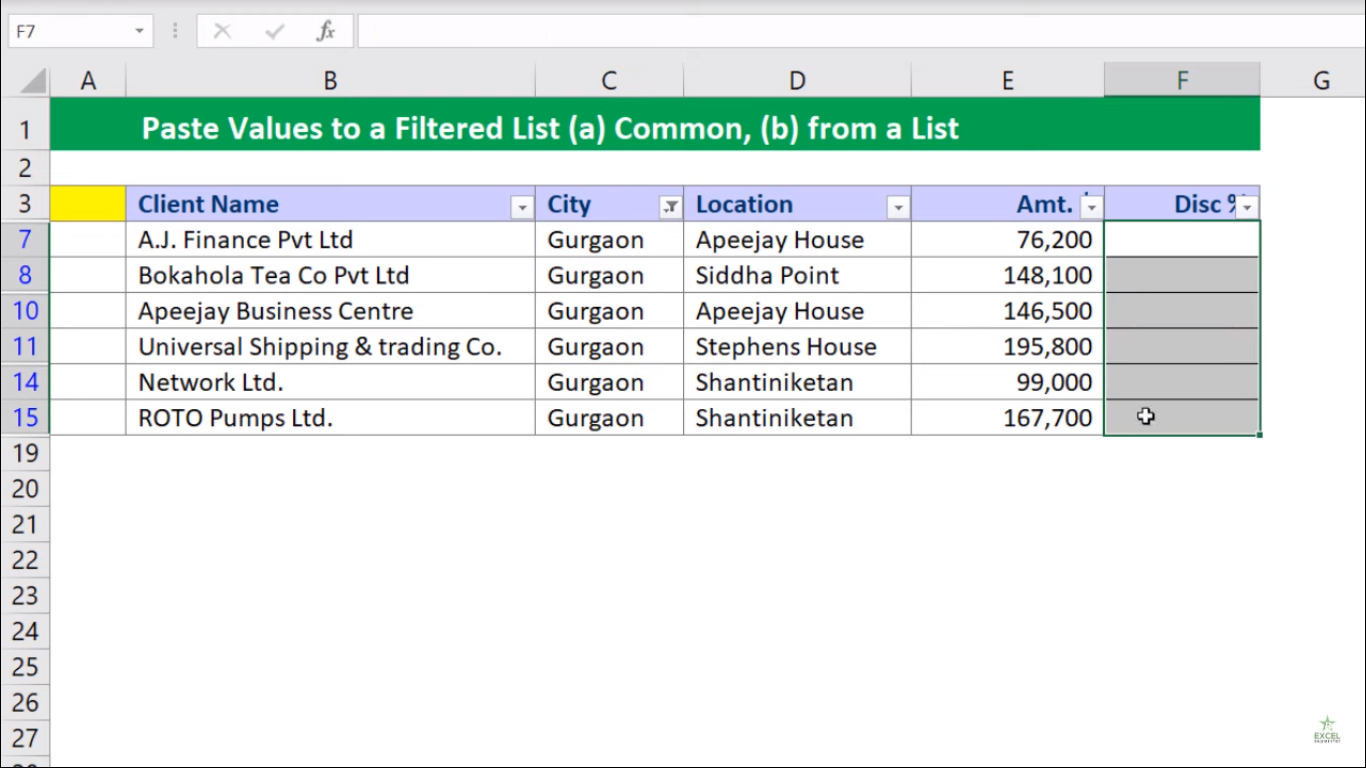1. Select the entire cells from the Discount Column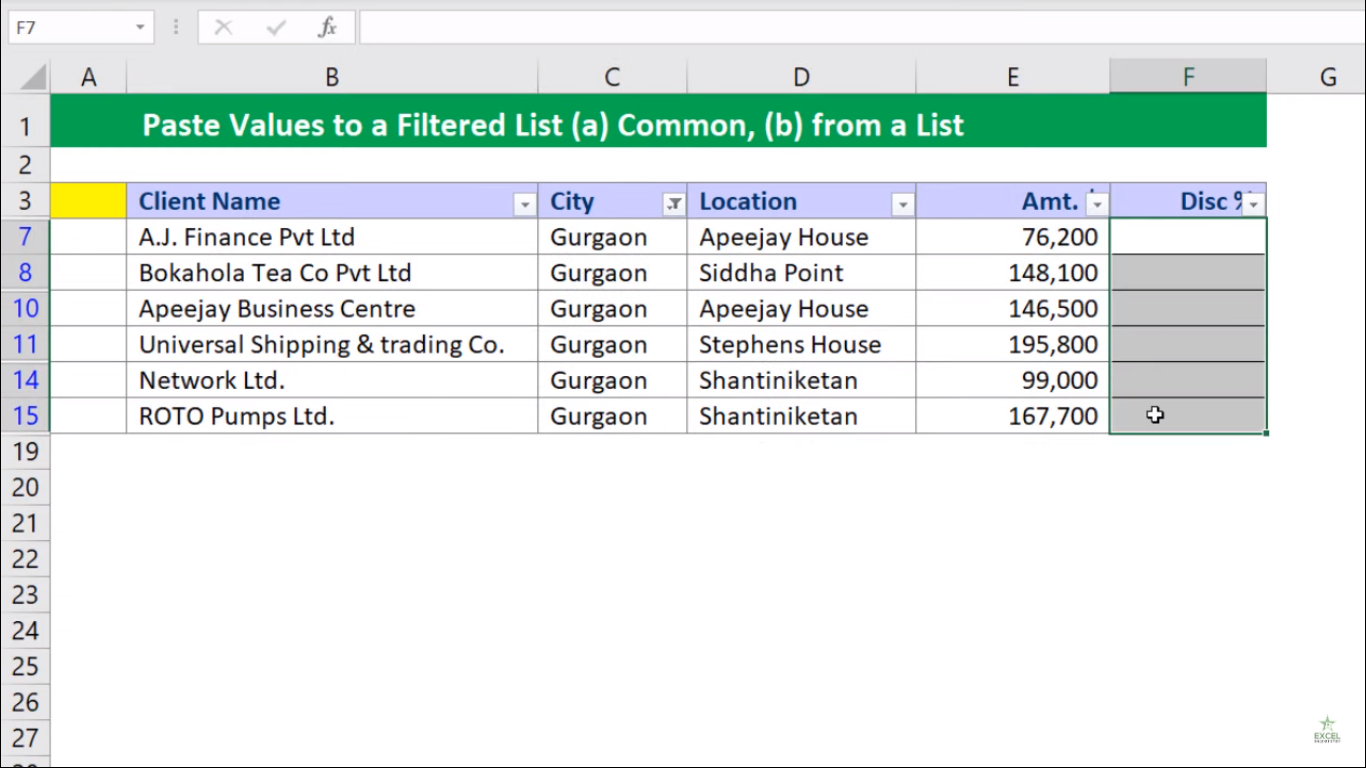2. Write Discount Percentage as 10% and press Ctrl + Enter Shortcut Key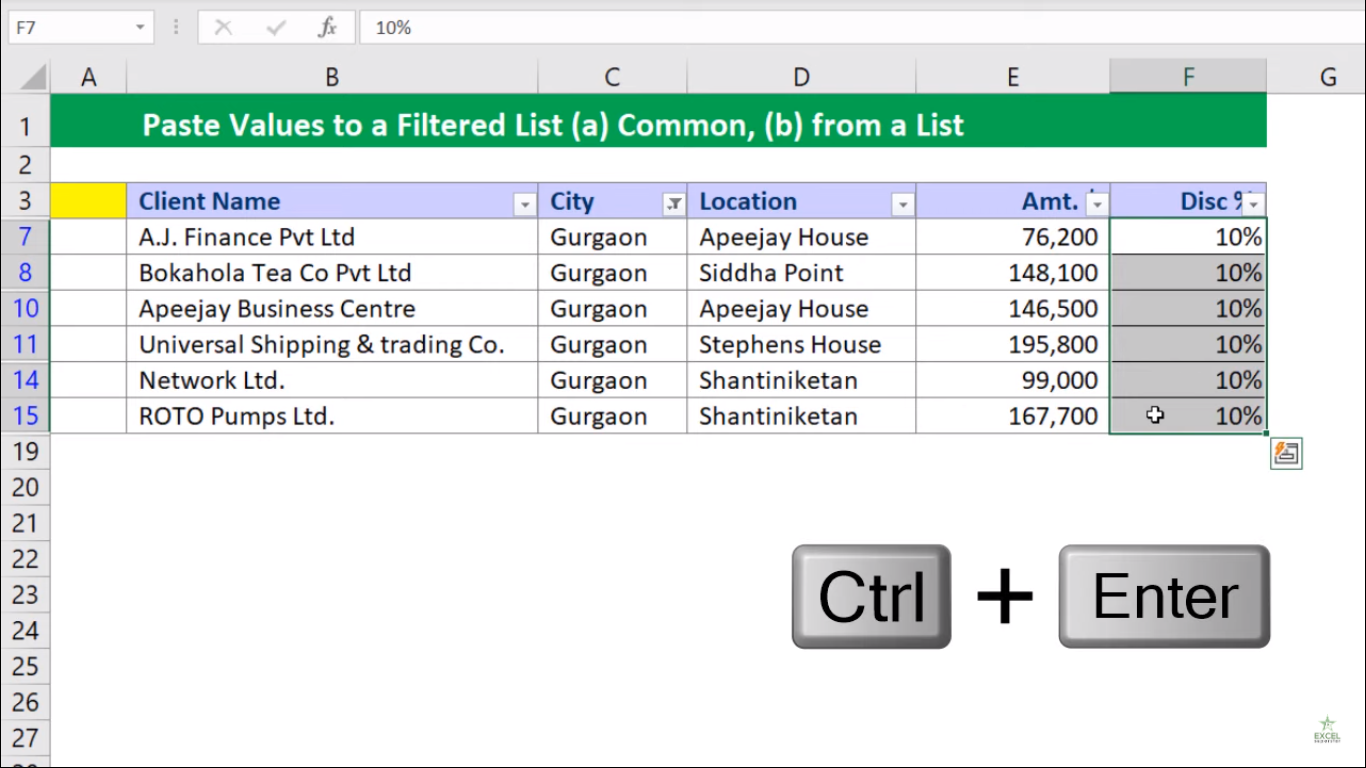3. Remove the Filter

4. Lastly you will see only those cells with 10% Discount in all the Gurgaon City Values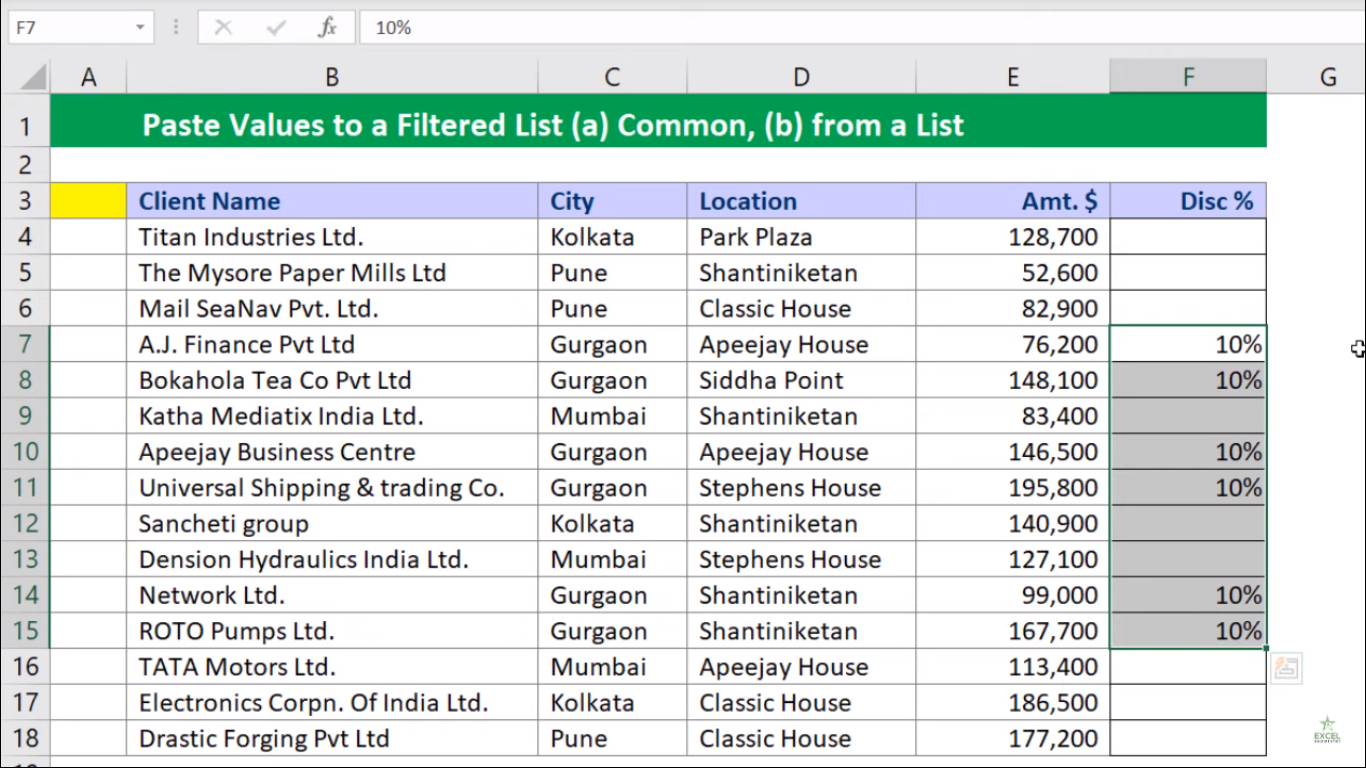2. From the List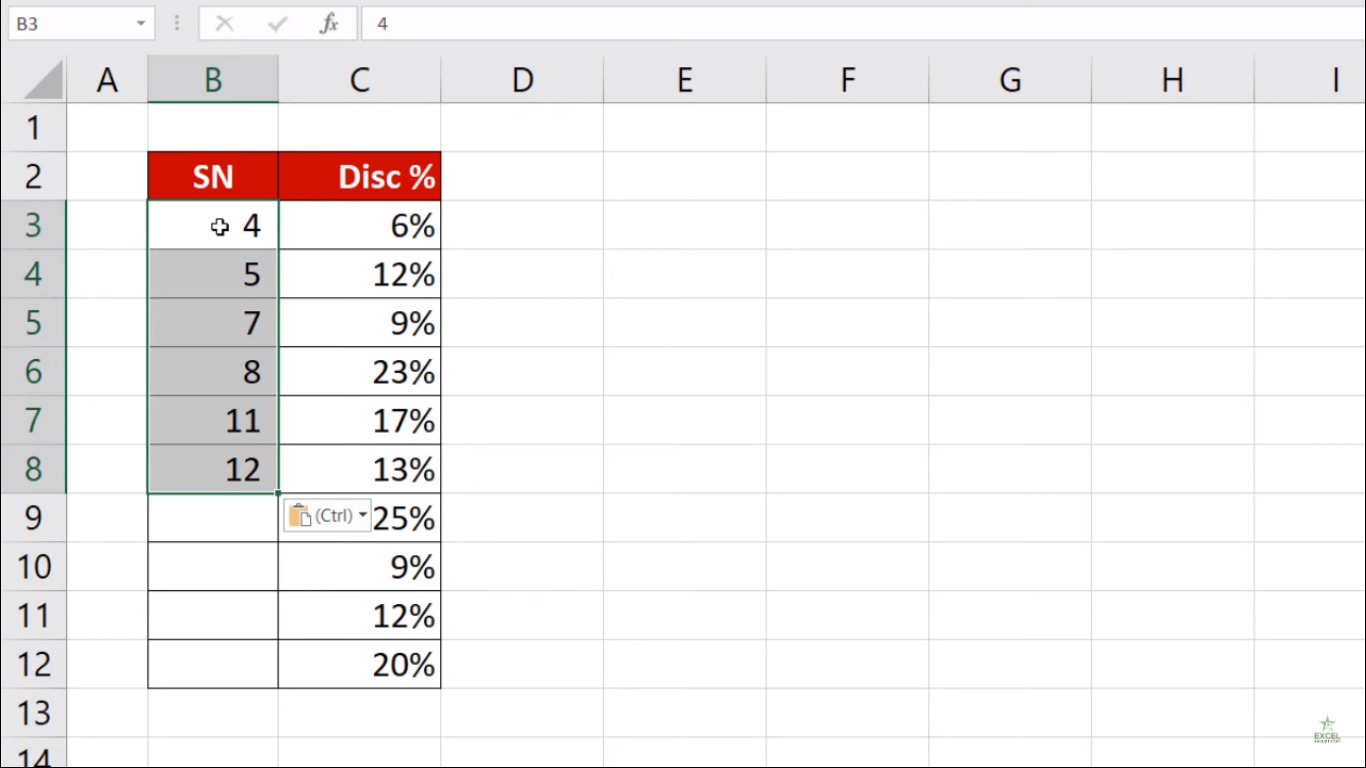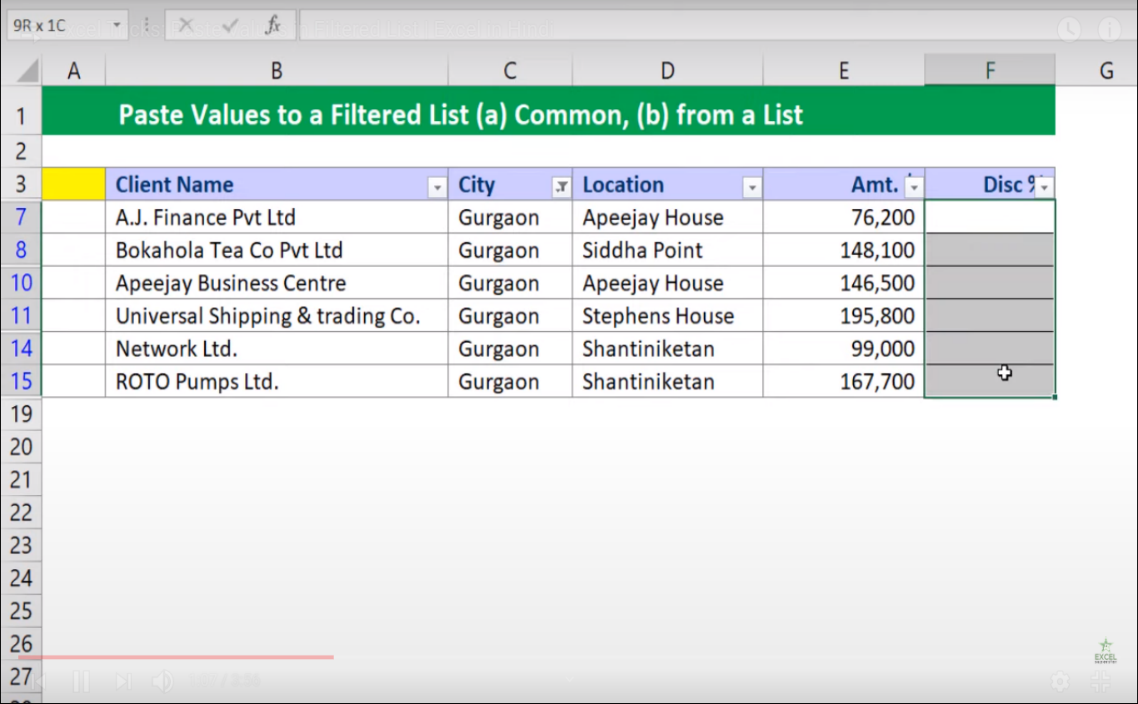Now here I have a second table and, I want that the first six values from the table should be applied in the Discount Column for all the Gurgaon city Values in the first table rather than a single Discount Percentage for all Gurgaon the City Values in the Discount Column. So here’s how you can do it:

1. Create a Serial Number at the start of the table

2. Enter number such as 1 and 2 and drag the number to the bottom of the table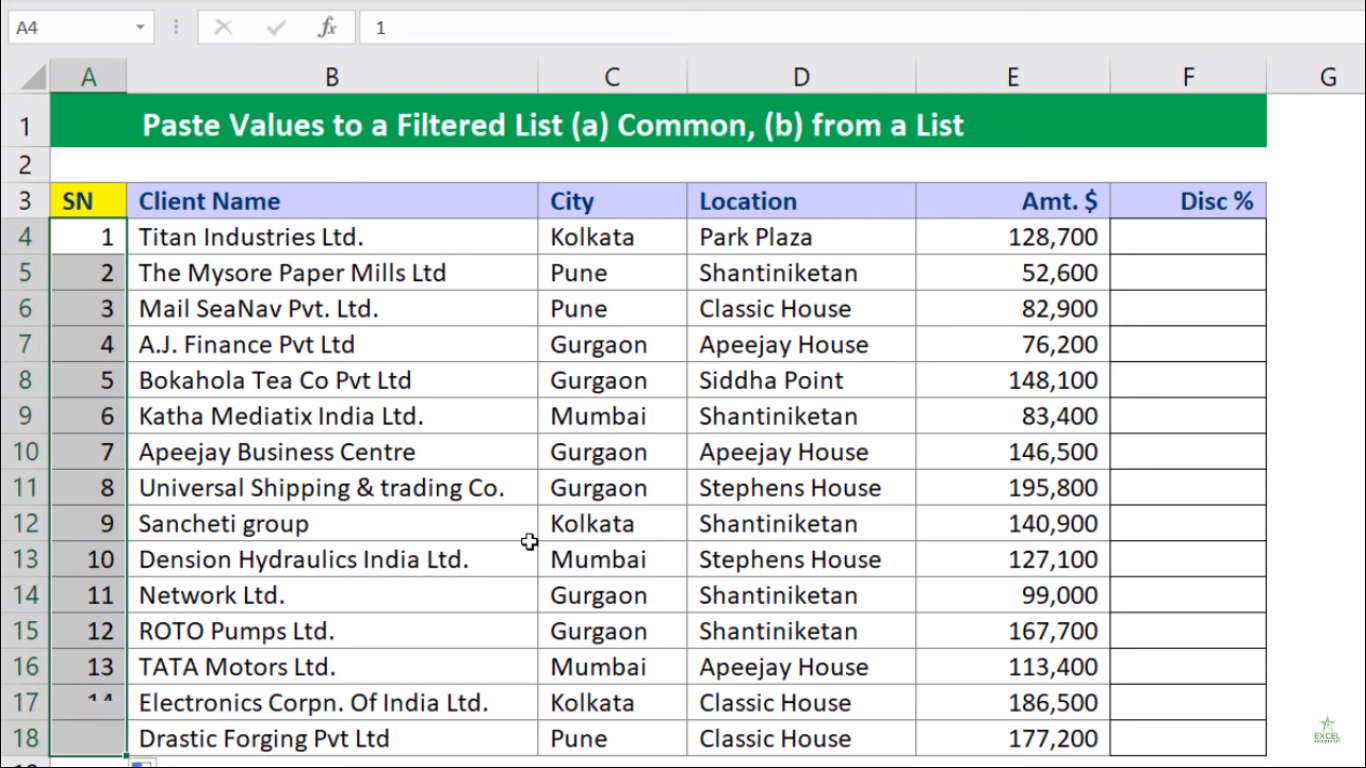3. Now apply the filter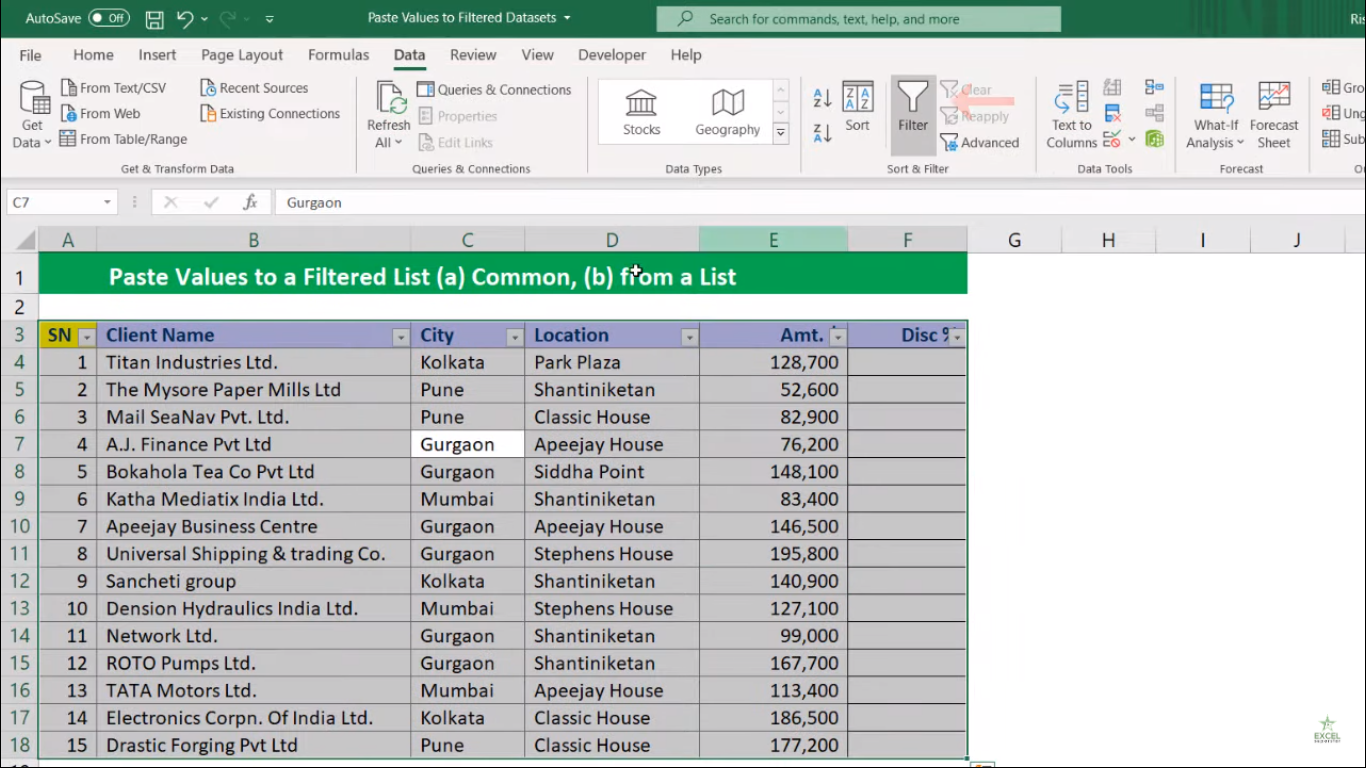4. Select the Gurgaon City from the City Column dropdown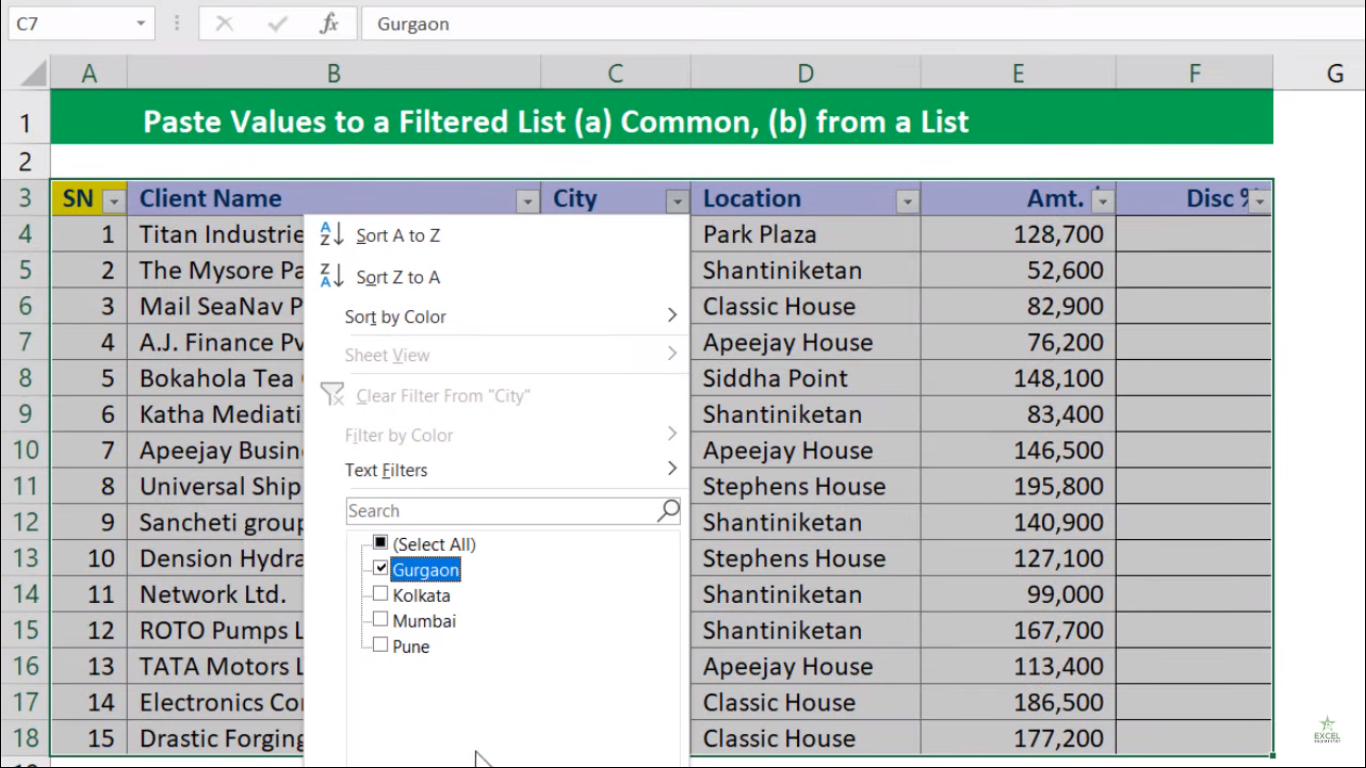5. Copy the Serial Number and paste into a different listNow here we will be applying VLOOKUP Formula

6. Press =VLOOKUP and open the bracket =VLOOKUP(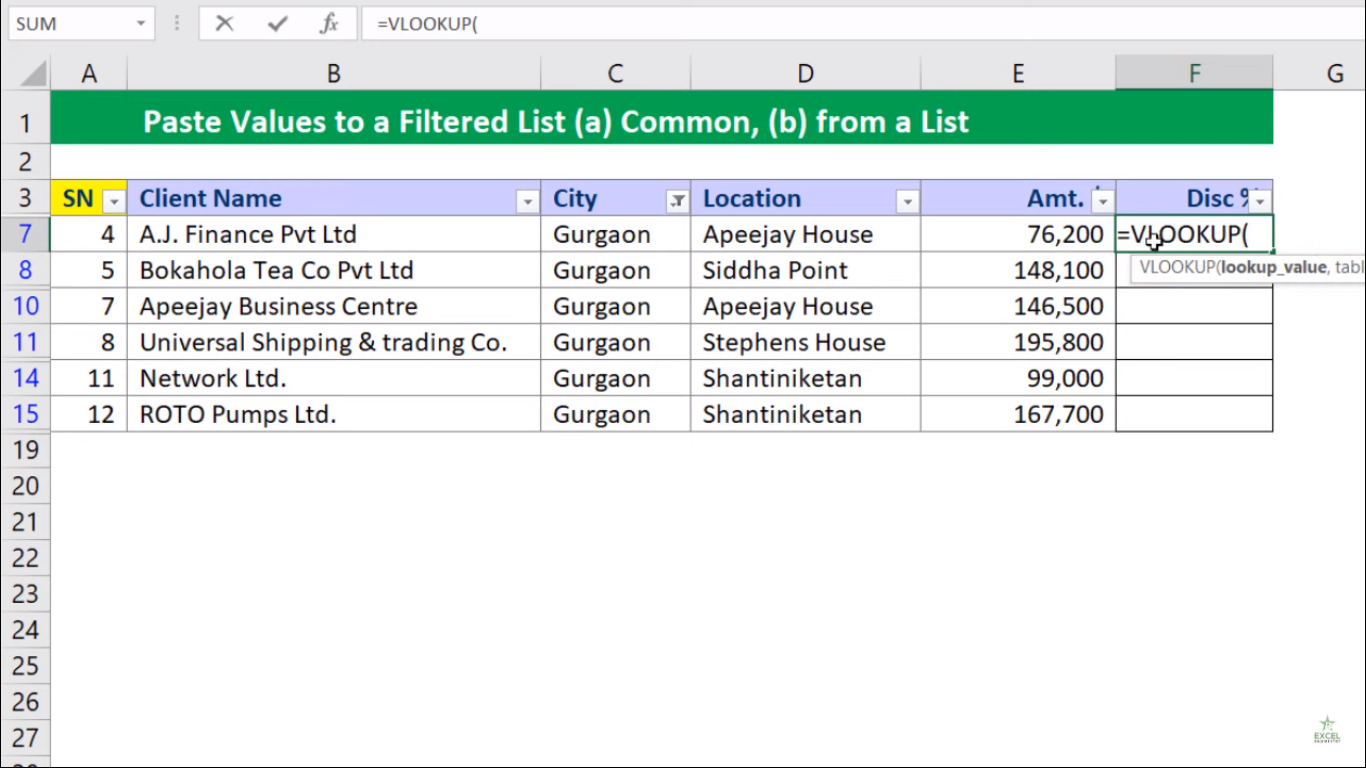Now here there 4 parameters (lookup_value, table_array, col_index_num, match mode)

7. Select the Serial Number 4 as lookup_value and press comma (,) =VLOOKUP(A7,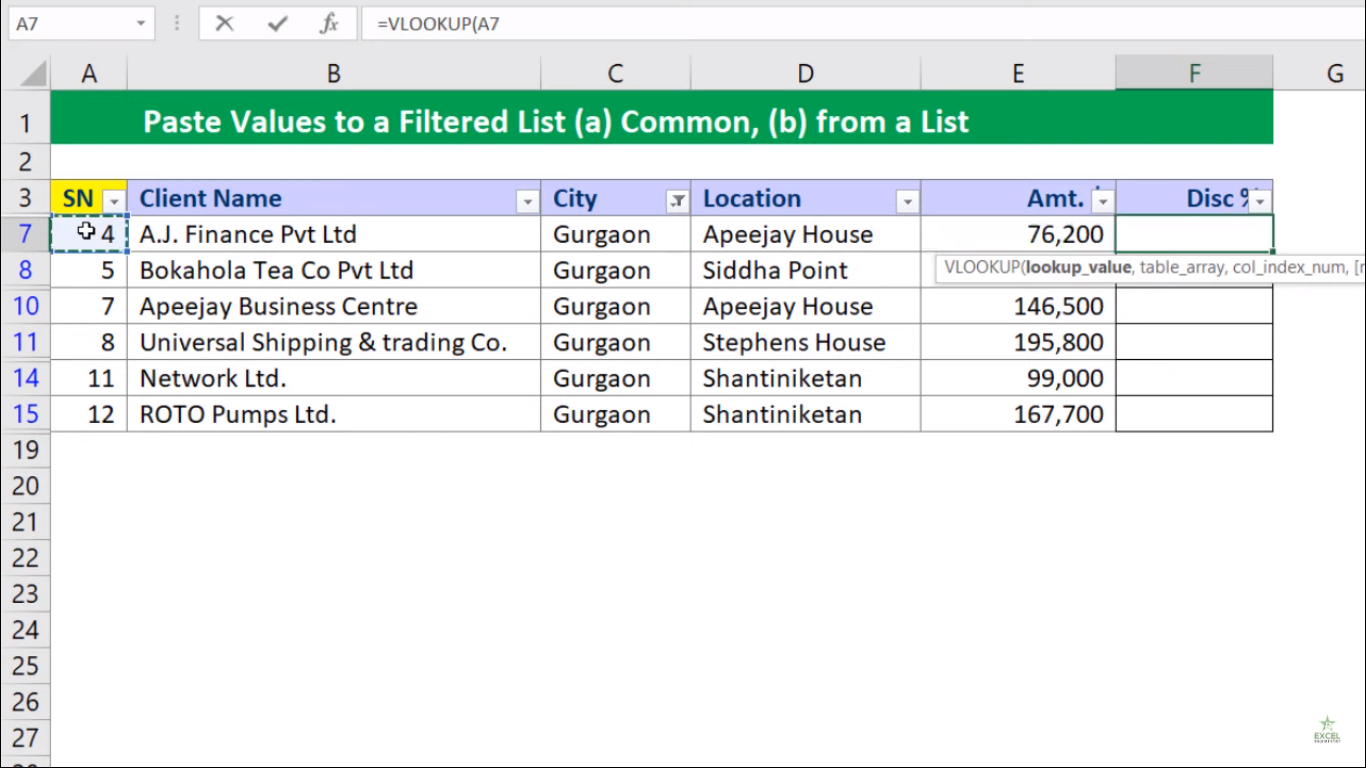8. Go to Next Sheet choose the entire table as table array and press F4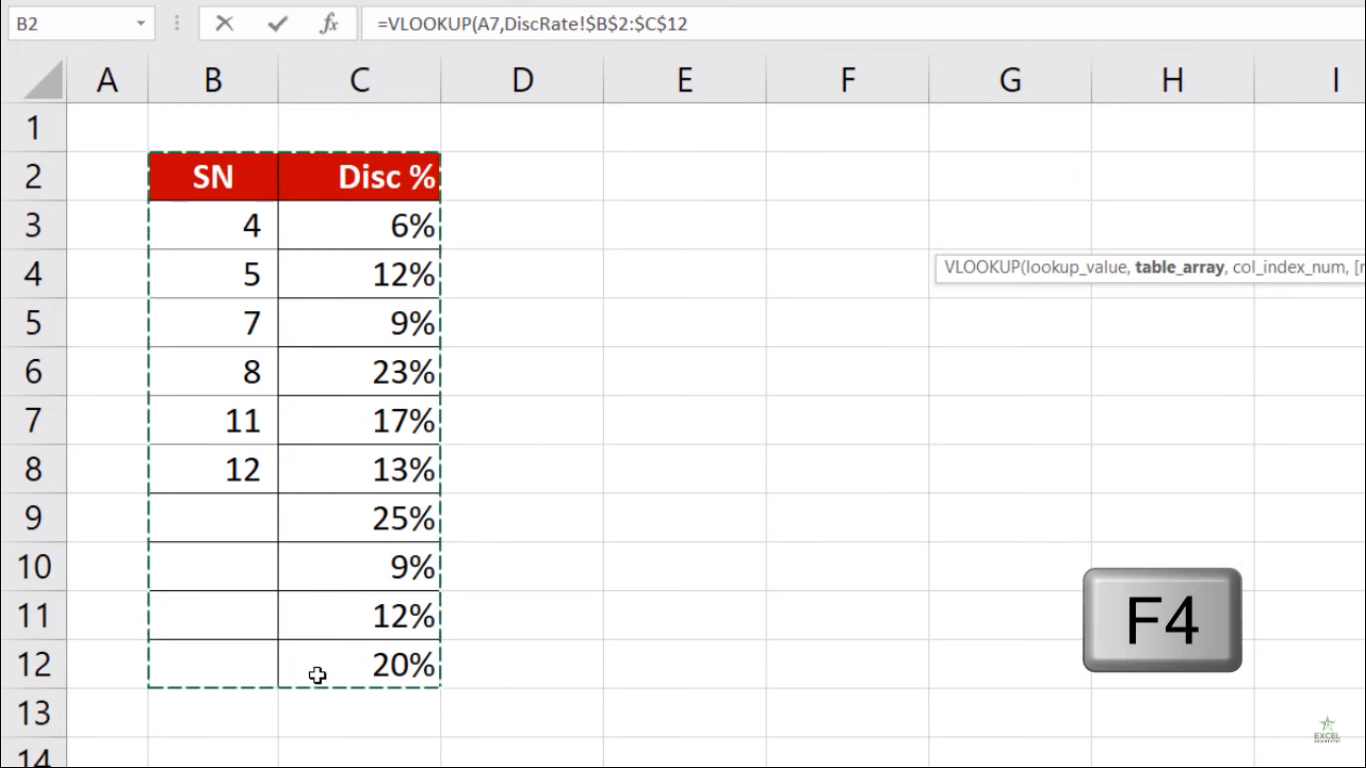9. Write 2 for col_index_num and 0 for Exact match and close the bracket =VLOOKUP(A7, 2,0)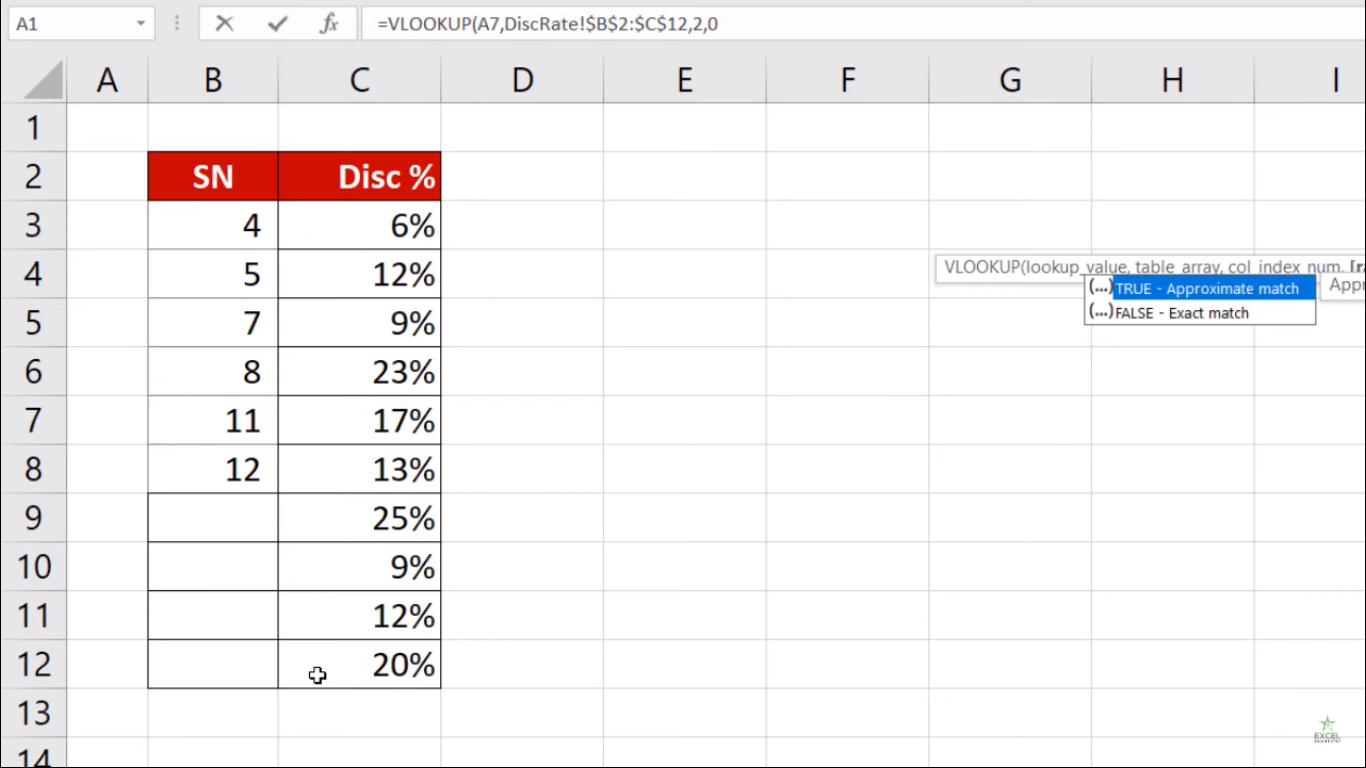10. Press Enter and copy the values and drag it to the bottom

11. Lastly you will see that data is ready for all the values of Gurgaon City inside the table